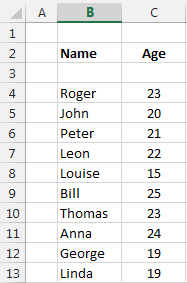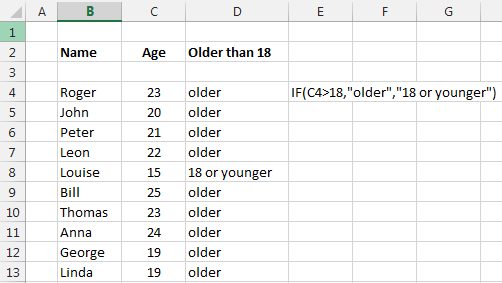## The IF function in Excel

The IF function checks whether a condition (or a set of conditions, when nested) is met, and returns one value if the condition is TRUE and another value if the condition is FALSE.

It allows you to create very complex formulas that can make your sheet do some extremely complex calculation.

Some easy examples, like checking who in a list of people is older than 18, or if a number is bigger or smaller than another.

=IF(test , value_if_TRUE , value_if_FALSE)

Lets check who in this list of people is older than 18.This is done by writing  = IF(CELL AGE > 18, "older", "junger")

Like in the following pictureSee how easy it is....

You can enter all the following signs as a condition

A<B      A is smaller than B

A>B      A is bigger/greater than B

A<=B    A is smaller or equal than B

A>=B    A is bigger or equal than B

A=B      A equals B

A<>B    A is different than B

You can also write function in the condition like

IF(A1*10)<=(A2/5), A1,A2)

This checks if A1*10 is smaller or equal than A2/5

In the result you can also enter a function or an operation like

IF(A<B,    A*10+B,    B*Sin(A)+RAND()*10-2)

Excel is very flexible so you can also avoid the IF statement just by typing formulas like this

=(A>B)*A*20% + (A<=B)*A*10%

In text this means

If the A value is bigger than B than the final value is equal to 20% of A

and

if the A value is smaller or equal than B, then the final value is equal to 10% of A.

The reason of this is because =(A>B) is a boolean expression with result 1 or 0 depending of A and B.

### BOOLEAN FUNCTIONS also called LOGICAL FUNCTIONS

One more chapter is to check the boolean AND, OR, NOT functions.

These are useful to compare many different result (must be numbers).

AND(condition1, condition 2, condition 3, ....): if all condition are true (1) then the result is TRUE or 1; or it can be seen as if only one is 0 or FALSE, then the result is false.

=AND(A1>A2,A1<A3)

OR(condition1, condition 2, condition 3, ....): if only ONE is TRUE (1), then the result is TRUE (1)

=OR(A1>A2,A1<A3)

NOT(condition): returns the opposite of the condition.

=NOT(A1>A2)

And you can combine them.

=AND(A1>A2,NOT(A1<A3))

### FALSE and TRUE

The FALSE and TRUE functions are use to set the value of a cell to False or True

in Cell A1  write =False  or just False.  You do not need the equal sign for this

If you want to test the condition of a cell, then write =if(A1=True, "write something true", "write something false")